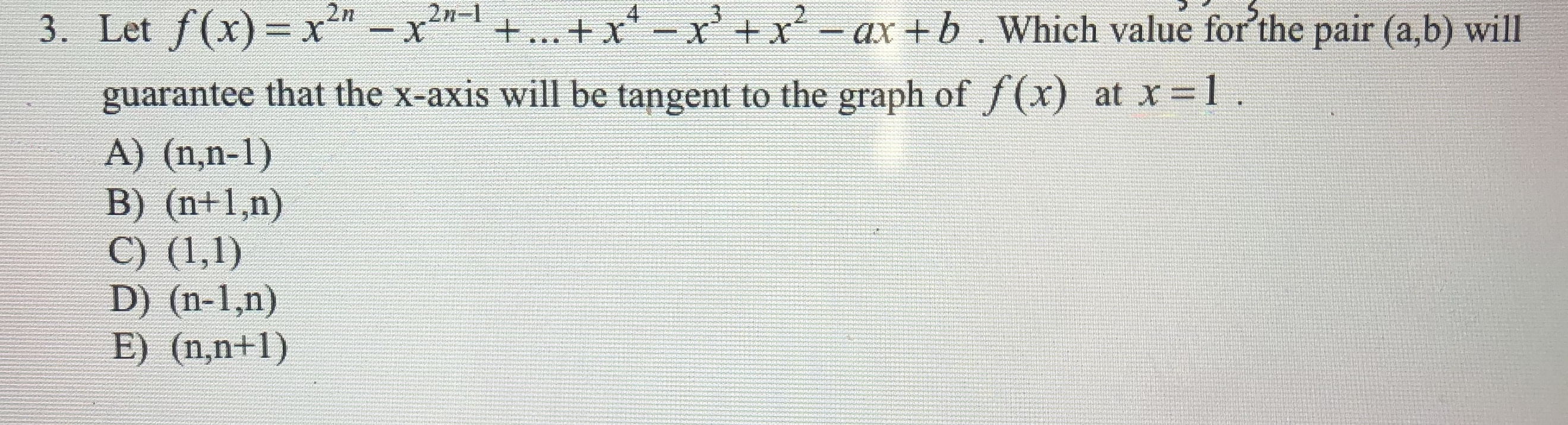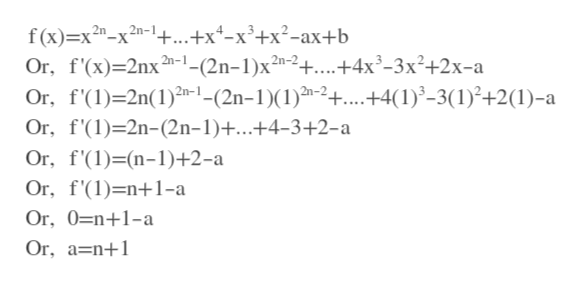# 2n-X2n3. Let f(x)- x-x+... + xxx-ax+b. Which value for'the pair (a,b) willXguarantee that the x-axis will be tangent to the graph of /(x)at x 1A) (n,n-1)B) (n+1,n)C) (1,1)D) (n-1,n)E) (n,n+1)

Question
9 viewshelp_outlineImage Transcriptionclose2n- X 2n 3. Let f(x)- x-x +... + xxx-ax+b. Which value for'the pair (a,b) will X guarantee that the x-axis will be tangent to the graph of /(x) at x 1 A) (n,n-1) B) (n+1,n) C) (1,1) D) (n-1,n) E) (n,n+1) fullscreen
check_circle

Step 1

At x=1 the graph of f(x) touches x axis. This means at x=1, f(x)=0

Or, f(1)=0

From it we get equation: a-b=1

Step 2

At x=1 tangent is x-axis, which is horizontal. So, f'(1)=0, because slop...help_outlineImage Transcriptionclosef (x)—х 2n-х 2n-1 +...+x*-x3+x?-ах+b Or, f'(x)-2nx Or, f(1)-2n(1)th-1-(2n-1)(1)-2+....+4(1)*-3(1)*+2(1)-а 2n- 1- (2n-1)x2n-2 +.+4x3-3х?+2х-а Or, f'(1)-2n-(2n-1)+...+4-3+2-а Or, f'(1)-[(n-1)+2-а Or, f(1)-n+1-а Or, 0=n+1-a Or, a n fullscreen

### Want to see the full answer?

See Solution

#### Want to see this answer and more?

Solutions are written by subject experts who are available 24/7. Questions are typically answered within 1 hour.*

See Solution
*Response times may vary by subject and question.
Tagged in

### Derivative